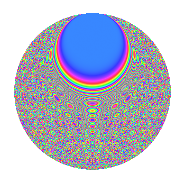# Properties

 Label 945.2.csLevel 945 Weight 2 Character orbit cs Rep. character $$\chi_{945}(104,\cdot)$$ Character field $$\Q(\zeta_{18})$$ Dimension 840 Newforms 2 Sturm bound 288 Trace bound 1

# Learn more about

## Defining parameters

 Level: $$N$$ = $$945 = 3^{3} \cdot 5 \cdot 7$$ Weight: $$k$$ = $$2$$ Character orbit: $$[\chi]$$ = 945.cs (of order $$18$$ and degree $$6$$) Character conductor: $$\operatorname{cond}(\chi)$$ = $$945$$ Character field: $$\Q(\zeta_{18})$$ Newforms: $$2$$ Sturm bound: $$288$$ Trace bound: $$1$$ Distinguishing $$T_p$$: $$2$$

## Dimensions

The following table gives the dimensions of various subspaces of $$M_{2}(945, [\chi])$$.

Total New Old
Modular forms 888 888 0
Cusp forms 840 840 0
Eisenstein series 48 48 0

## Trace form

 $$840q$$ $$\mathstrut -\mathstrut 24q^{4}$$ $$\mathstrut -\mathstrut 30q^{9}$$ $$\mathstrut +\mathstrut O(q^{10})$$ $$840q$$ $$\mathstrut -\mathstrut 24q^{4}$$ $$\mathstrut -\mathstrut 30q^{9}$$ $$\mathstrut -\mathstrut 30q^{11}$$ $$\mathstrut -\mathstrut 6q^{14}$$ $$\mathstrut +\mathstrut 24q^{21}$$ $$\mathstrut -\mathstrut 12q^{25}$$ $$\mathstrut +\mathstrut 12q^{29}$$ $$\mathstrut +\mathstrut 78q^{30}$$ $$\mathstrut -\mathstrut 36q^{35}$$ $$\mathstrut +\mathstrut 12q^{36}$$ $$\mathstrut -\mathstrut 108q^{39}$$ $$\mathstrut -\mathstrut 36q^{44}$$ $$\mathstrut -\mathstrut 12q^{46}$$ $$\mathstrut +\mathstrut 6q^{49}$$ $$\mathstrut +\mathstrut 24q^{50}$$ $$\mathstrut -\mathstrut 60q^{51}$$ $$\mathstrut +\mathstrut 120q^{56}$$ $$\mathstrut -\mathstrut 150q^{60}$$ $$\mathstrut -\mathstrut 348q^{64}$$ $$\mathstrut -\mathstrut 18q^{65}$$ $$\mathstrut +\mathstrut 21q^{70}$$ $$\mathstrut -\mathstrut 36q^{71}$$ $$\mathstrut -\mathstrut 48q^{74}$$ $$\mathstrut -\mathstrut 60q^{79}$$ $$\mathstrut +\mathstrut 54q^{81}$$ $$\mathstrut +\mathstrut 150q^{84}$$ $$\mathstrut -\mathstrut 42q^{85}$$ $$\mathstrut -\mathstrut 192q^{86}$$ $$\mathstrut -\mathstrut 6q^{91}$$ $$\mathstrut -\mathstrut 210q^{95}$$ $$\mathstrut +\mathstrut 12q^{99}$$ $$\mathstrut +\mathstrut O(q^{100})$$

## Decomposition of $$S_{2}^{\mathrm{new}}(945, [\chi])$$ into irreducible Hecke orbits

Label Dim. $$A$$ Field CM Traces $q$-expansion
$$a_2$$ $$a_3$$ $$a_5$$ $$a_7$$
945.2.cs.a $$24$$ $$7.546$$ $$\Q(\sqrt{-35})$$ $$0$$ $$0$$ $$0$$ $$0$$
945.2.cs.b $$816$$ $$7.546$$ None $$0$$ $$0$$ $$0$$ $$0$$